# scatterhistogram

Create scatter plot with histograms

## Syntax

``scatterhistogram(tbl,xvar,yvar)``
``scatterhistogram(tbl,xvar,yvar,'GroupVariable',grpvar)``
``scatterhistogram(xvalues,yvalues)``
``scatterhistogram(xvalues,yvalues,'GroupData',grpvalues)``
``scatterhistogram(___,Name,Value)``
``scatterhistogram(parent,___)``
``s = scatterhistogram(___)``

## Description

example

````scatterhistogram(tbl,xvar,yvar)` creates a scatter plot with marginal histograms from the table `tbl`. The `xvar` input indicates the table variable to display along the x-axis. The `yvar` input indicates the table variable to display along the y-axis.```

example

````scatterhistogram(tbl,xvar,yvar,'GroupVariable',grpvar)` uses the table variable specified by `grpvar` to group observations specified by `xvar` and `yvar`.```

example

````scatterhistogram(xvalues,yvalues)` creates a scatter plot of the data in `xvalues` and `yvalues` and displays the marginal histograms for the `xvalues` and `yvalues` data along the x-axis and y-axis, respectively.```

example

````scatterhistogram(xvalues,yvalues,'GroupData',grpvalues)` uses the data in `grpvalues` to group the data in `xvalues` and `yvalues`.```

example

````scatterhistogram(___,Name,Value)` specifies additional options for the scatter plot with marginal histograms using one or more name-value pair arguments. Specify the options after all other input arguments. For a list of properties, see ScatterHistogramChart Properties.```
````scatterhistogram(parent,___)` creates the scatter plot with marginal histograms in the figure, panel, or tab specified by `parent`.```

example

````s = scatterhistogram(___)` returns the `ScatterHistogramChart` object. Use `s` to modify the object after you create it. For a list of properties, see ScatterHistogramChart Properties.```

## Examples

collapse all

Create a scatter plot with marginal histograms from a table of data for medical patients.

Load the `patients` data set and create a table from a subset of the variables loaded into the workspace. Then, create a scatter histogram chart comparing the H`eight` values to the W`eight` values.

```load patients tbl = table(LastName,Age,Gender,Height,Weight); s = scatterhistogram(tbl,'Height','Weight');```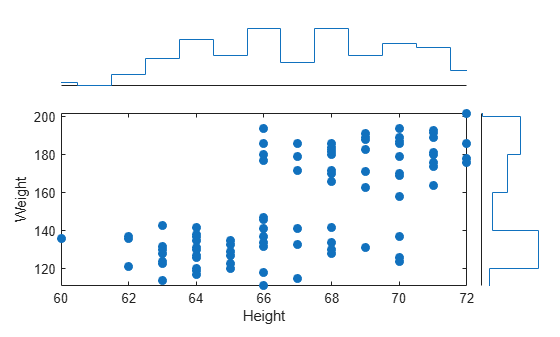Using the `patients` data set, create a scatter plot with marginal histograms and specify the table variable to use for grouping the data.

Load the `patients` data set and create a scatter histogram chart from the data. Compare the patients' `Systolic` and `Diastolic` values. Group the data according to the patients' smoker status by setting the `'GroupVariable'` name-value pair argument to `'Smoker'`.

```load patients tbl = table(LastName,Diastolic,Systolic,Smoker); s = scatterhistogram(tbl,'Diastolic','Systolic','GroupVariable','Smoker');```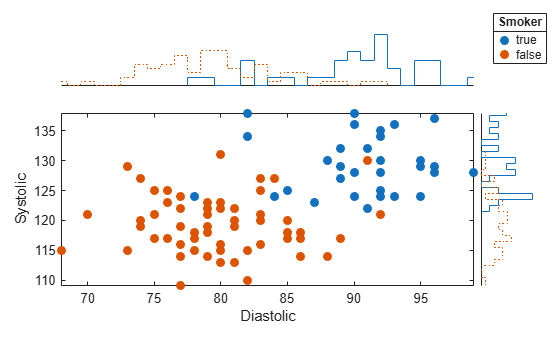Use a scatter plot with marginal histograms to visualize categorical and numeric medical data.

Load the `patients` data set, and convert the `Smoker` data to a categorical array. Then, create a scatter histogram chart that compares patients' `Age` values to their smoker status. The resulting scatter plot contains overlapping data points. However, the y-axis marginal histogram indicates that there are far more nonsmokers than smokers in the data set.

```load patients Smoker = categorical(Smoker); s = scatterhistogram(Age,Smoker); xlabel('Age') ylabel('Smoker')```Create a scatter plot with marginal histograms using arrays of shoe data. Group the data according to shoe color, and customize properties of the scatter histogram chart.

Create arrays of data. Then, create a scatter histogram chart to visualize the data. Use custom labels along the x-axis and y-axis to specify the variable names of the first two input arguments. You can specify the title, axis labels, and legend title by setting properties of the `ScatterHistogramChart` object.

```xvalues = [7 6 5 6.5 9 7.5 8.5 7.5 10 8]; yvalues = categorical({'onsale','regular','onsale','onsale', ... 'regular','regular','onsale','onsale','regular','regular'}); grpvalues = {'Red','Black','Blue','Red','Black','Blue','Red', ... 'Red','Blue','Black'}; s = scatterhistogram(xvalues,yvalues,'GroupData',grpvalues); s.Title = 'Shoe Sales'; s.XLabel = 'Shoe Size'; s.YLabel = 'Price'; s.LegendTitle = 'Shoe Color';```

Change the colors in the scatter histogram chart to match the group labels. Change the histogram bin widths to be the same for all groups.

```s.Color = {'Red','Black','Blue'}; s.BinWidths = 1;```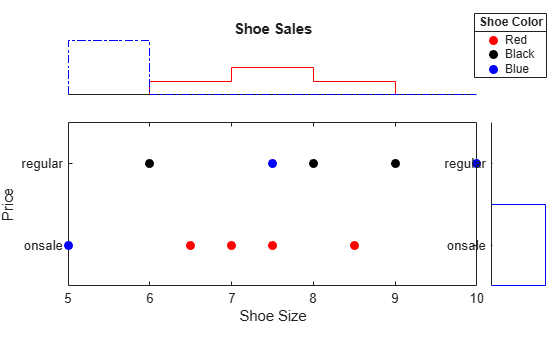Create a scatter plot with marginal histograms. Specify the number of bins and line widths of the histograms, the location of the scatter plot, and the legend visibility.

Load the `patients` data set and create a scatter histogram chart from the data. Compare the patients' `Diastolic` and `Systolic` values, and group the data according to the patients' `SelfAssessedHealthStatus` values. Adjust the histograms by specifying the `NumBins` and `LineWidth` options. Place the scatter plot in the `'NorthEast'` location of the figure by using the `ScatterPlotLocation` option. Ensure the legend is visible by specifying the `LegendVisible` option as `'on'`.

```load patients tbl = table(LastName,Diastolic,Systolic,SelfAssessedHealthStatus); s = scatterhistogram(tbl,'Diastolic','Systolic','GroupVariable','SelfAssessedHealthStatus', ... 'NumBins',4,'LineWidth',1.5,'ScatterPlotLocation','NorthEast','LegendVisible','on');```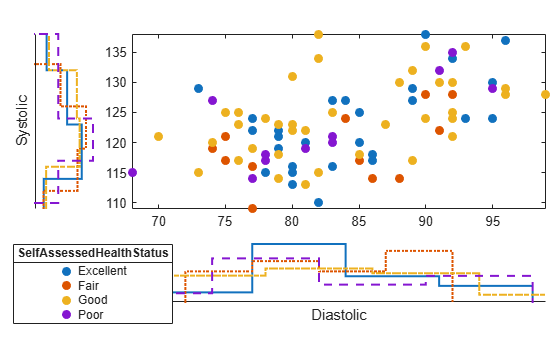Create a scatter plot with marginal histograms. Group the data by using a combination of two different variables.

Load the `patients` data set. Combine the `Smoker` and `Gender` data to create a new variable. Create a scatter histogram chart that compares the `Diastolic` and `Systolic` values of the patients. Use the new variable `SmokerGender` to group the data in the scatter histogram chart.

```load patients [idx,genderStatus,smokerStatus] = findgroups(string(Gender),string(Smoker)); SmokerGender = strcat(genderStatus(idx),"-",smokerStatus(idx)); s = scatterhistogram(Diastolic,Systolic,'GroupData',SmokerGender,'LegendVisible','on'); xlabel('Diastolic') ylabel('Systolic')```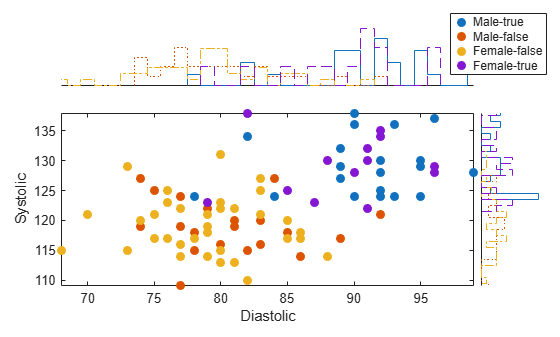Create a scatter plot with kernel density marginal histograms. This example requires a Statistics and Machine Learning Toolbox™ license.

Load the `carsmall` data set and create a scatter histogram chart from the data. Compare the `Horsepower` and `MPG` values. Use the number of cylinders to group the data by setting the `GroupVariable` option to `Cylinders`. Specify kernel density histograms by setting the `HistogramDisplayStyle` option to `'smooth'`. Specify a solid line for all the histograms by setting the `LineStyle` option to `'-'`.

```load carsmall tbl = table(Horsepower,MPG,Cylinders); s = scatterhistogram(tbl,'Horsepower','MPG', ... 'GroupVariable','Cylinders','HistogramDisplayStyle','smooth', ... 'LineStyle','-');```## Input Arguments

collapse all

Source table, specified as a table.

You can create a table from workspace variables using the `table` function, or you can import data as a table using the `readtable` function.

The `SourceTable` property of the `ScatterHistogramChart` object stores the source table.

Table variable for x-axis, specified in one of these forms:

• Character vector or string scalar — Indicating one of the variable names. For example, `scatterhistogram(tbl,'Acceleration','Horsepower')` selects the variable named `'Acceleration'` for the x-axis.

• Numeric scalar — Indicating the table variable index. For example, `scatterhistogram(tbl,5,3)` selects the fifth variable in the table for the x-axis.

• Logical vector — Containing one `true` element.

The values associated with your table variable must be of a numeric type or `categorical`.

The `XVariable` property of the `ScatterHistogramChart` object stores the selected variable name.

Table variable for y-axis, specified in one of these forms:

• Character vector or string scalar — Indicating one of the variable names. For example, `scatterhistogram(tbl,'Acceleration','Horsepower')` selects the variable named `'Horsepower'` for the y-axis.

• Numeric scalar — Indicating the table variable index. For example, `scatterhistogram(tbl,5,3)` selects the third variable in the table for the y-axis.

• Logical vector — Containing one `true` element.

The values associated with your table variable must be of a numeric type or `categorical`.

The `YVariable` property of the `ScatterHistogramChart` object stores the selected variable name.

Table variable for grouping data, specified in one of these forms:

• Character vector or string scalar — Indicating one of the variable names

• Numeric scalar — Indicating the table variable index

• Logical vector — Containing one `true` element

The values associated with your table variable must form a numeric vector, logical vector, categorical array, string array, or cell array of character vectors.

`grpvar` splits the data in `xvar` and `yvar` into unique groups. Each group has a default color and an independent histogram in each axis. In the legend, `scatterhistogram` displays the group names in order of their first appearance in `GroupData`.

Example: `'Model_Year'`

Example: `2`

Values appearing along the x-axis, specified as a numeric vector or categorical array.

The `XData` property of the `ScatterHistogramChart` object stores the `xvalues` data.

Example: `[0.5 4.3 2.4 5.6 3.4]`

Example: `categorical({'small','medium','small','large','medium','small'})`

Values appearing along the y-axis, specified as a numeric vector or categorical array.

The `YData` property of the `ScatterHistogramChart` object stores the `yvalues` data.

Example: `[0.5 4.3 2.4 5.6 3.4]`

Example: `categorical({'small','medium','small','large','medium','small'})`

Group values for the scatter plot and the corresponding marginal histograms, specified as a numeric vector, logical vector, categorical array, string array, or cell array of character vectors.

`grpvalues` splits the data in `xvalues` and `yvalues` into unique groups. Each group has a default color and an independent histogram in each axis. In the legend, `scatterhistogram` displays the group names in order of their first appearance in `GroupData`.

Example: `[1 2 1 3 2 1 3]`

Example: `categorical({'blue','green','green','blue','green'})`

Parent container, specified as a `Figure`, `Panel`, `Tab`, `TiledChartLayout`, or `GridLayout` object.

### Name-Value Pair Arguments

Specify optional comma-separated pairs of `Name,Value` arguments. `Name` is the argument name and `Value` is the corresponding value. `Name` must appear inside quotes. You can specify several name and value pair arguments in any order as `Name1,Value1,...,NameN,ValueN`.

Example: `scatterhistogram(tbl,xvar,yvar,'GroupVariable',grpvar,'HistogramDisplayStyle','stairs')` specifies `grpvar` as the grouping variable and displays stairstep plots next to the scatter plot.

Note

The properties listed here are only a subset. For a complete list, see ScatterHistogramChart Properties.

Chart title, specified as a character vector, string array, cell array of character vectors, or categorical array. The default chart has no title.

To create a multiline title, specify a string array or cell array of character vectors. Each element in the array corresponds to a line of text.

If you specify the title as a categorical array, MATLAB® uses the values in the array, not the categories.

Example: ```s = scatterhistogram(__,'Title','My Title Text')```

Example: `s.Title = 'My Title Text'`

Example: `s.Title = {'My','Title'}`

Number of histogram bins, specified as a positive integer scalar, 2-by-1 positive integer vector, or 2-by-n positive integer matrix, where n is the number of groups in `GroupData`.

Specified ValueDescription
scalarThe value is the number of bins for the x and y histograms.
2-by-1 vectorThe first value is the number of bins for the x data, and the second value is the number of bins for the y data.
2-by-n matrixThe `(1,j)` value is the number of bins for the histogram of the x data that is in the `j`th group. Similarly, the `(2,j)` value is the number of bins for the histogram of the y data that is in the `j`th group.

`scatterhistogram` uses the `'BinMethod','auto'` name-value pair argument of `histogram` to determine the default `NumBins` and `BinWidths` values.

You cannot change `NumBins` for categorical data.

Example: ```s = scatterhistogram(__,'NumBins',20)```

Example: `s.NumBins = [10; 15]`

Histogram display style, specified as one of these options.

Display StyleDescription
`'stairs'`Display a stairstep plot that shows the outline of the histogram without filling the bars.
`'bar'`Display a histogram bar plot.
`'smooth'`Display a smooth plot generated through kernel density estimates. This option requires a Statistics and Machine Learning Toolbox license.

`scatterhistogram` uses the `'pdf'` type of normalization to generate the histograms. For more information, see the `'Normalization'` name-value pair argument of `histogram`.

Example: ```s = scatterhistogram(__,'HistogramDisplayStyle','smooth')```

Example: `s.HistogramDisplayStyle = 'bar'`

Histogram line width, specified as a positive scalar or positive vector in points. By default, `scatterhistogram` assigns a line width of `0.5` to each histogram plot line.

When the total number of groups exceeds the number of specified line widths, `scatterhistogram` cycles through the specified line widths.

Example: `s = scatterhistogram(__,'LineWidth',0.75)`

Example: `s.LineWidth = [0.5 0.75 0.5]`

Marker symbol for each scatter plot group, specified in one of these forms:

• Character vector designating a marker style

• String array or cell array of character vectors designating one or more marker styles

Choose among these marker options.

ValueDescription
`'o'`Circle
`'+'`Plus sign
`'*'`Asterisk
`'.'`Point
`'x'`Cross
`'_'`Horizontal line
`'|'`Vertical line
`'square'` or `'s'`Square
`'diamond'` or `'d'`Diamond
`'^'`Upward-pointing triangle
`'v'`Downward-pointing triangle
`'>'`Right-pointing triangle
`'<'`Left-pointing triangle
`'pentagram'` or `'p'`Five-pointed star (pentagram)
`'hexagram'` or `'h'`Six-pointed star (hexagram)
`'none'`No markers

By default, `scatterhistogram` assigns the marker symbol `'o'` to each group in the scatter plot. When the total number of groups exceeds the number of specified symbols, `scatterhistogram` cycles through the specified symbols.

Example: `s = scatterhistogram(__,'MarkerStyle','x')`

Example: `s.MarkerStyle = {'x','o'}`

Location of the scatter plot, specified as one of these options.

LocationDescription
`'SouthWest'`Plot the histograms above and to the right of the scatter plot.
`'SouthEast'`Plot the histograms above and to the left of the scatter plot.
`'NorthEast'`Plot the histograms below and to the left of the scatter plot.
`'NorthWest'`Plot the histograms below and to the right of the scatter plot.

Example: ```s = scatterhistogram(__,'ScatterPlotLocation','NorthEast')```

Example: `s.ScatterPlotLocation = 'SouthEast'`

State of legend visibility, specified as `'on'` or `'off'`. Set `LegendVisible` to `'on'` to display the legend or `'off'` to hide the legend.

If `GroupData` is empty (`[]`) or contains a single group, then `scatterhistogram` does not display a legend. Otherwise, `scatterhistogram` displays a legend by default, unless the legend overlaps the scatter plot or marginal histograms.

In the legend, `scatterhistogram` displays the group names in order of their first appearance in `GroupData`.

Example: `s = scatterhistogram(__,'LegendVisible','on')`

Example: `s.LegendVisible = 'off'`

## Output Arguments

collapse all

`ScatterHistogramChart` object, which is a standalone visualization. Use `s` to modify the object after you create it. For a list of properties, see ScatterHistogramChart Properties.

collapse all

### Standalone Visualization

A standalone visualization is a chart designed for a special purpose that works independently from other charts. Unlike other charts such as `plot` and `surf`, a standalone visualization has a preconfigured axes object built into it, and some customizations are not available. A standalone visualization also has these characteristics:

• It cannot be combined with other graphics elements, such as lines, patches, or surfaces. Thus, the `hold` command is not supported.

• The `gca` function can return the chart object as the current axes.

• You can pass the chart object to many MATLAB functions that accept an axes object as an input argument. For example, you can pass the chart object to the `title` function.

## Tips

• To interactively explore the data in your `ScatterHistogramChart` object, use these options. Some of these options are not available in the Live Editor.

• Zoom/pan — Use the scroll wheel or the + and - buttons to zoom. Click and drag the scatter plot to pan. `scatterhistogram` updates the marginal histograms based on the data within the current scatter plot limits.

• Data tips — Hover over the scatter plot or marginal histograms to display a data tip.

• If you create a scatter plot with marginal histograms from a table, then you can customize data tips for the scatter plot.

• To add or remove a row from the data tip, right-click anywhere on the scatter plot and point to Modify Data Tips. Then, select or deselect a variable.

• To add or remove multiple rows, right-click on the plot, point to Modify Data Tips, and select More. Then, add variables by clicking or remove them by clicking .

### Topics

Introduced in R2018b